Tech Interview

# Top 35+ Data Structures Interview Questions And Answers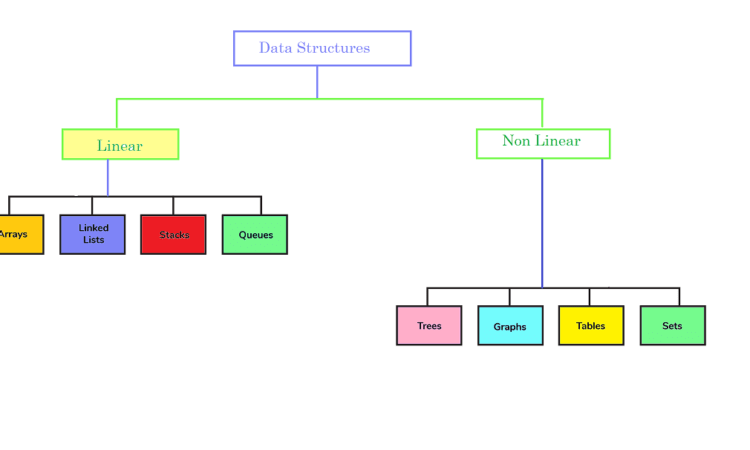### What is Data Structure?

• Data structure is an essential concept in any programming language and it is vital for algorithmic design.
• It’s used to efficiently organize and modify data.
• DS refers to how data and relationships are represented. It helps determine how efficient various operations or algorithms can work.

### Types of Data Structure

• There are two types data structures.
• Linear data structure: A linear data structure is one that results in a sequence of elements or a linear listing. Example: Arrays, Linked List, Stacks, Queues etc.
• Non-linear data structures: A non-linear structure is one where the elements of the data structure result in an arrangement that does not allow for sequential traversal of nodes. Example: Trees, Graphs etc.

### Applications

Data structures are the fundamental basis of software programming, as the most efficient algorithm for tackle a particular problem relies on how well a given data is organized.

• Identifiers lookups within compilers implementations have been constructed by using hash tables.
• The B-trees data structures are appropriate for databases.
• The most significant areas in which the data structure can be used are:
1. Artificial intelligence
2. Compiler design
3. Machine learning
4. Design and management of databases
5. Blockchain
6. The Numerical as well as Statistical analysis
7. Operating system development
8. Image & Speech Processing
9. Cryptography

### Benefits of learning Data Structures

Each problem has limitations regarding how quickly the issue must be solved (time) and the amount of less resources the issue consumes(space). In other words, a challenge is constrained by size and complexity of the time within which it needs to be dealt with efficiently.

• To accomplish this, it’s very crucial for the issue to be presented in a structured manner on which efficient algorithms can be implemented.
• Selecting the correct data structure is the first and most crucial step prior to applying any algorithm to any issue.

#### Data structures are written in C, Java

• The fundamental concepts behind data structures remain the same in all programming languages. The implementation is different in accordance with the syntax or nature of the structure used by the programming language.
• The implementation of procedural languages such as C is accomplished by using pointsers, structures, etc.
• In a language that is object-oriented such as Java data structures are constructed using objects and classes.
• A thorough understanding of the basic concepts behind every data structure will help you to stand out in interviews since selecting the appropriate data structure is the first step in solving the issue effectively.

## Data structure Interview Questions

#### 1.Can you please explain the differences between file structure and storage?

• File Structure: Data representation into secondary or additional memory. Any device, such as pen drives or hard disks that store data that is not deleted manually or which does not lose any data, is known as a “file structure representation”.
• Storage Structure: This type stores data in the main memory, i.e RAM. Once the function using this data finishes, it is erased.
• This is because storage structure stores data in the memory of the computer, while file structure stores data in the auxiliary memory.

#### 2.Are you able to tell the difference between non-linear and linear data structures?

• A linear data structure is one where the elements of a data structure result in a sequence, or a linear list. In non-linear data formats, however, traversal of nodes occurs in a nonlinear fashion.
• Lists, queues, stacks and queues are examples for linear data structures, whereas graphs or trees are examples of nonlinear data structures.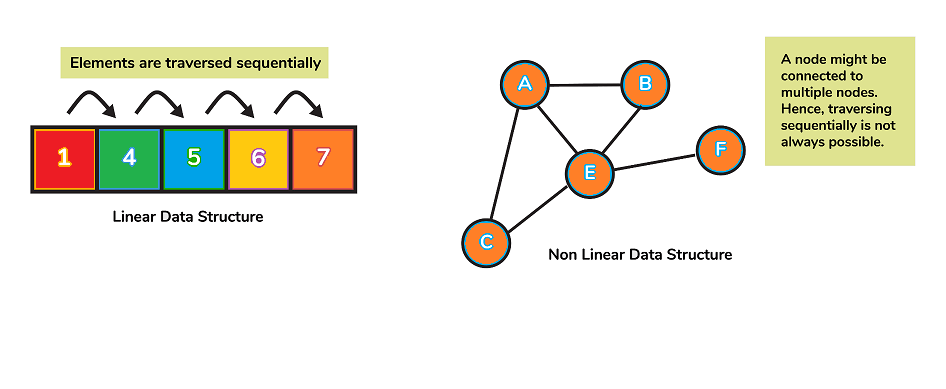#### 3.What is an array?

• Arrays are a collection of similar data that is stored at contiguous storage locations.
• This is the simplest type of data structure, where each data element can be accessed randomly by simply using its index number.

#### 4.What is a multidimensional array and how does it work?

• Multi-dimensional arrays refer to data structures that span more than one dimension.
• This means that more than one index variable will be needed for each point of storage. This data structure is used when data cannot be stored or represented using one dimension. 2D arrays are the most common multidimensional arrays.
• 2D arrays mimic the tabular structure. They are easy to store the bulk of the data that can be accessed using row or column pointers.#### 5.What is a link list?

A linked list is a data format that contains nodes, where each node is connected to another node using a reference pointer. They are and not stored in adjacent memories. To form a chain, they are linked with pointers. This creates a link chain-like for data storage.

• There are two parts to each node element:
• a data field
• A reference (or pointer to) the next node.
• The head is the first node of a linked list. NULL is the last node. The reference field contains null to indicate that the last node is the one. If the list is empty, then the head is null.

#### 6.Is it linear or non-linear?

Linked lists can be viewed as both linear and nonlinear data structures. It all depends on the purpose of linked lists.

• A linked list can be used to access strategies. It is considered a linear data structure. It can also be used to store data.

#### 7.What makes linked lists more efficient that arrays?

1. Insertion and deletion
• In an array, inserting and deleting elements is costly as there must be enough space to accommodate them.
• However, updating an address in a linked listing is much easier because we only need to update the address in the next pointer.
2. Dynamic Data Structure
• A linked list is a dynamic data format that doesn’t require an initial size to be given at creation. It can grow or shrink at runtime through allocating and dealinglocating memory.
• However, an array’s size is limited by the number of items that are statically stored in its main memory.
3. Memory is not lost
• Because it is allotted in runtime, the linked list’s size can change depending on its needs.
• If we declare an array with 10 elements and store 3 elements, it is wasted space. Therefore, arrays are more susceptible to memory wastage.

#### 8.Describe the situations where linked lists and arrays can be used.

• These are some examples of situations where linked list is used over array.
• If we don’t know the exact number of elements.
• We know there will be many add-or-remove operations.
• There are fewer random access operations.
• The linked list is better if you want to insert items in the middle of a list. This would be useful when creating priority queues.
• Here are some examples of cases in which arrays can be used over the linked list.
• We need to index or randomly gain elements more often.
• It is easier to allocate the correct amount of memory if we already know how many elements are in an array.
• We need to be fast while we iterate over the elements of the sequence.
• Memory is a problem
• Because of the nature and purpose of linked lists and arrays, filled arrays are less memory-intensive than linked lists.
• Each element of the array represents only the data, while each linked list node represents both the data and one or more pointers to other elements in that linked list.
• When deciding on which data structure to use over others, space, time and ease of implementation must all be considered.

#### 9.What is a doubly linked list (DLL).What are its uses?

• This is a complicated type of linked list in which a node has at least two references.
• One that connects with the next node in the sequence
• Another one that connects with the previous node.
• This structure allows for traversal of data elements in both directions (left-to-right and vice versa).
• DLL applications are:
1. A music playlist that includes next and previous song navigation options.
2. The browser cache using BACK-FORWARD was used to visit pages
3. You can use the undo and redo functions on platforms like word, paint, etc. to reverse the node to go to the previous page.

#### 10.What is a stack?What are the uses of stack?

• Stack is a linear data format that uses LIFO (Last in First Out) to access elements.
• The basic operations of a stack are push, pop, top, or peek.
• Here are some examples of stack applications:
1. In an expression, check for balanced parentheses
2. Evaluation of a postfix expression
3. Problem with Infix to Postfix Conversion
4. Reverse a string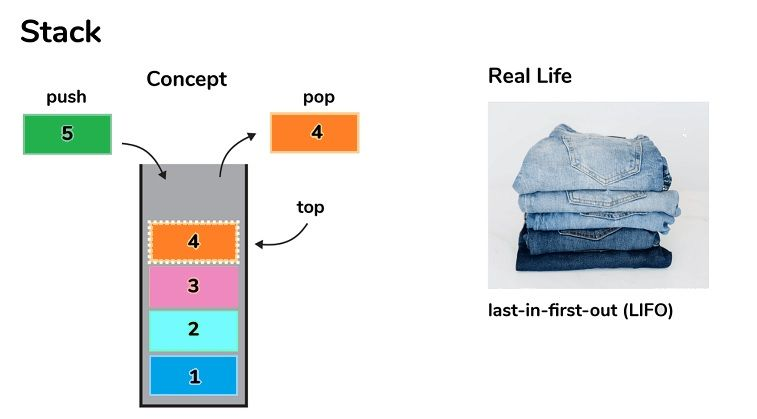#### 11.What is the meaning of a queue?What are the potential uses of queue?

• The term “queue” refers to a type of linear data structure which follows its FIFO (First In, First Out) method of accessing the elements.
• Dequeue in the queue, enqueue the element in the queue obtain the front part of the queue and finally, get the rear part of the queue are some of the basic tasks which can be carried out.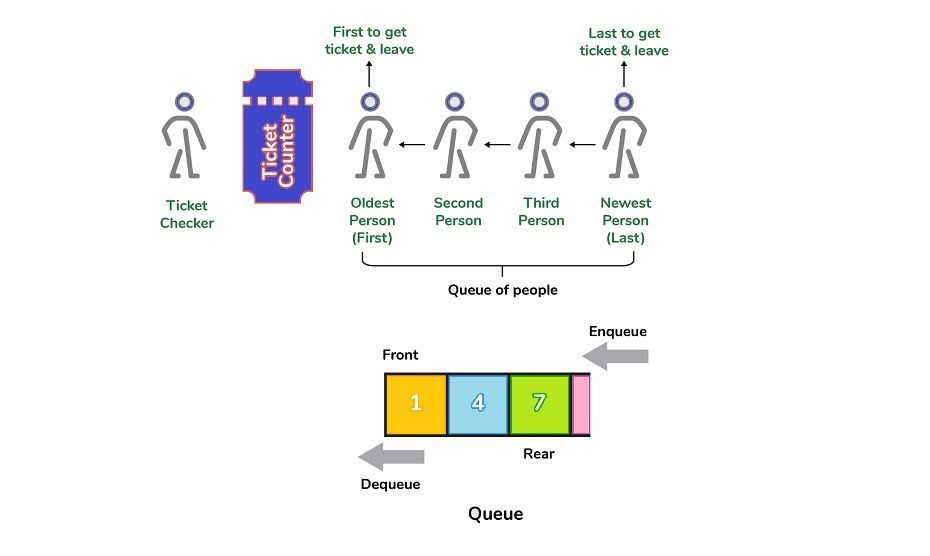• Some of the uses of queue include:
2. BFS algorithm to determine the shortest distance between two points in graph.
3. Processing of requests for website on the internet
4. As buffers in programs such as MP3 music player, DVD player etc.
5. Controlling an input stream

#### 12.What makes a stack different from an actual queue?

• In a stack in a stack, the item most recently added to the stack is removed first. In queues, the item that was that was added the least first.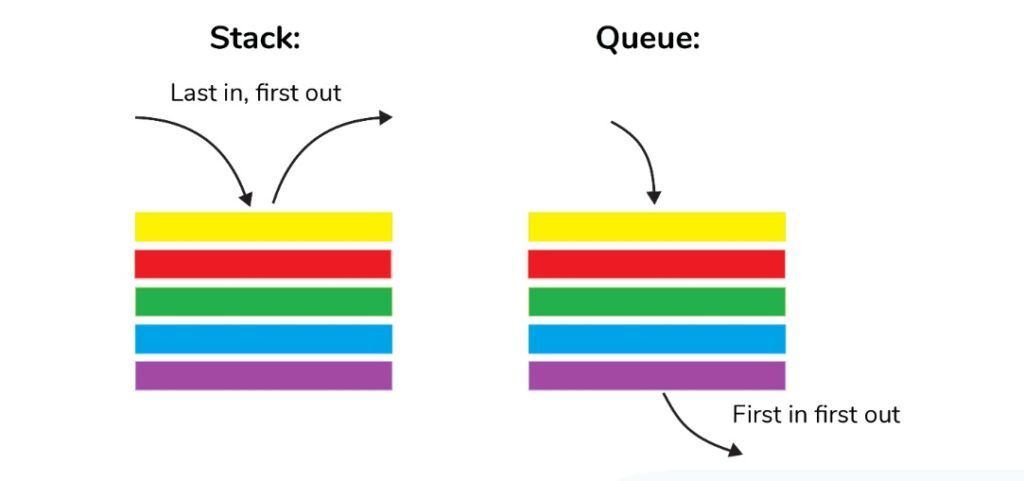#### 13.Define the procedure behind the process of storing variables in memory.

• Variables are stored within memory according to how much memory required. Here are the steps in storing a variable
• The memory amount required is allocated first.
• Then, it’s stored according to the data structure utilized.
• Utilizing concepts like dynamic allocation can ensure high efficiency and ensures that storage units are accessed depending on the needs in real-time.

#### 14.How do you implement an order queue with stack?

• A queue can be created by using 2 stacks. Let `the q` become the queue, and `stack1` and `stack2` are the two stacks used to implement `the q`. It is known that stack can support pop, push, and other operations. To make use of these operations it is necessary to mimic the queue’s operations which is to enqueue as well as dequeue. Therefore the queue `q` can be implemented using two ways (Both methods make use of the auxillary complexity of space O(n)):

In order to make enqueue operations more expensive:

• In this case, the oldest element always is in the topmost position the `stack1` which allows dequeue operation to be completed within O(1) timing complexity.
• To put element on highest of the stack1, stack2 can be utilized.
• Pseudocode:
• Enqueue: Here, the time the complexity is O(n)
```enqueue(q, data):
While stack1 is not empty:
Push everything from stack1 to stack2.
Push data to stack1
Push everything back to stack1.```

Dequeue: Here, the time complex will equal O(1)

```deQueue(q):
If stack1 is empty then error
else
Pop an item from stack1 and return it```

In order to make dequeue operation more expensive:

• In this case, in order to enqueue the operation the new element is placed at the very top `the stack1`. In this case, the enqueue duration is O(1).
• If dequeue is empty, `the stack` has been empty for a while, the elements of `the stack 1` are transferred into `the stack2` and the top of `stack2` is the result. Simply, it is reversing the list is done by pushing into a stack, and then returning the first enqueued item. This process to push all the elements into a new stacks takes O(n) complex.
• Pseudocode:
• Enqueue: Time complexity: O(1)
```enqueue(q, data):
Push data to stack1```

Dequeue: Time complexity: O(n)

```dequeue(q):
If both stacks are empty then raise error.
If stack2 is empty:
While stack1 is not empty:
push everything from stack1 to stack2.
Pop the element from stack2 and return it.```

#### 15.How do you implement stack using queues?

• A stack is implemented with two queues. We are aware that a queue can support the enqueue as well as dequeue operation. Utilizing these functions, we have to create push, pop and other operations.
• Let stack be’s as well as the queues that are used to implement it be “q1” and “q2”. In the end, stack’s is executed in two methods:
1. In order to make push operations more expensive:
• This technique ensures that the any newly entered element will always be in front of ‘q1’. So the pop operation only dequeues from the first element, ‘q1’..
• “q2” is used as an secondary queue that puts each new element in ahead of ‘q1’ and making sure that pop occurs in O(1) complex.
• Pseudocode:
• The push element stacks up Then push is O(n) the time complex
• ```push(s, data):
Enqueue data to q2
Dequeue elements one by one from q1 and enqueue to q2.
Swap the names of q1 and q2
```
• Pop element from stack s: Takes O(1) time complexity.
```pop(s):
dequeue from q1 and return it.```

The pop operation is costly because it costs money:

• In a push operation the element is queued to q1.
• In the pop operation, all elements of q1, excluding the last element left are transferred into q2 if empty. The final element left of q1 gets dequeued and returned.
• Pseudocode:
• Push element is stacked on top of s Then push has O(1) the time-complexity
• ```push(s,data):
Enqueue data to q1
```
• Pop element from stack s: Takes O(n) time complexity.
```pop(s):
Step1: Dequeue every elements except the last element from q1 and enqueue to q2.
Step2: Dequeue the last item of q1, the dequeued item is stored in result variable.
Step3: Swap the names of q1 and q2 (for getting updated data after dequeue)
Step4: Return the result.```

#### 16.What is hashmap in the data structure?

• Hashmap is a type of data structure that makes use of the tables that allows data access in continuous timing (O(1)) and with a high degree of complexity provided you have the key.

#### 17.What is the prerequisite that an object be utilized as a key to or value within HashMap?

• The value or key object that is used in the hashmap should incorporate `equals()` and `hashcode()` method.
• This code can be utilized to insert an object that is key into the map. The equals method is utilized to extract information on the map.

#### 18.How Does HashMap manage collisions with Java?

• It is the `java.util.HashMap` class in Java employs the technique of chaining to deal with collisions. When chaining, if new values using the similar keys are pushed, be pushed, the values are saved in a linked lists stored in the bucket of the key in an element of a chain, alongside the value that is already present.
• In the worst-case scenario, it could happen that all keys have the same hashcode. This could cause the hash table changing into linked lists. In this scenario the process of searching for a value will require O(n) complexity, as instead of O(1) time because of its nature as a linked list. Thus, care should be taken when choosing a the hashing algorithm.

#### 19.What is the time-complexity of simple operations?() and then put() in the HashMap class?

• Time complexity of O(1) assuming that the hash function employed in the hash map is distributed evenly across buckets.

#### 20.What data structures are utilized to implement LRU cache?

• LRU cache, also known as Least Recently Used cache is a the quick identification of elements that hasn’t been put into usage for the longest period of duration by arranging the things in order of their usage. To achieve this, two different data structures are utilized:
• Queue – This is achieved by using a doubly-linked list. It is determined the maximum capacity of the queue by amount of cache space, i.e by the total amount of frames available. The pages that are least frequently used will be at the front of the queue, whereas the most frequently visited pages will be at the back of the queue.
• Hashmap Hashmap Hashmap records pages as keys, along with an address for the queue node in the form of.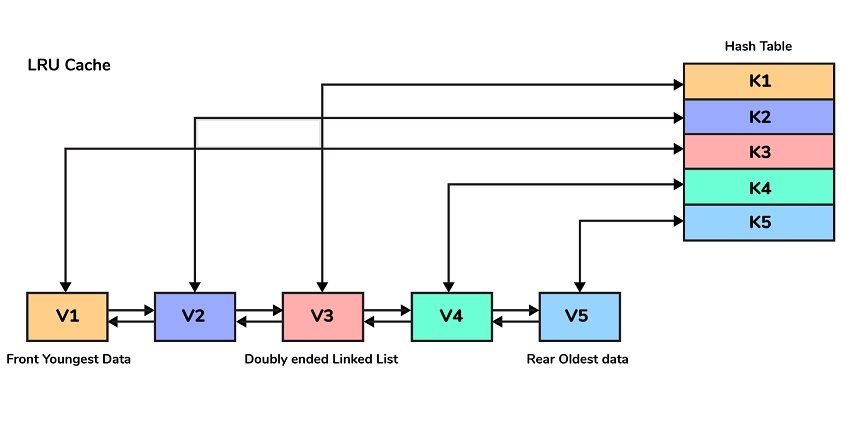#### 21.What is the definition of a priority queue?

• Priority queues are abstract data type similar to a normal queue however it has priority assigned to the elements.
• Priorities are given to elements that are processed prior to the elements with lower priority.
• In order to make this work you will require a minimum of two queues are needed to store the data, and another to keep the prioritization.

#### 22.Do we have the ability to store a double key within HashMap?

• No It is not possible to duplicate keys included in HashMap. If you attempt to insert any entry that has an existing key the previous value will be replaced with that new one. This will not alter the size of the HashMap.
• This is the reason keysetter() procedure returns every key as the SET method in Java because it does not permit duplicate keys.

#### 23.What is a data tree structure?

• The Tree can be described as a recursive non-linear structure of data that comprises the collection of data nodes, where one node is referred to as the root while the other nodes are referred to as descendants of this root.
• Tree organizes data into hierarchial manner.
• The most widely utilized Tree data structure that is most commonly used is binary trees as well as its variations.
• A few of the uses of trees include:
1. Filesystems files inside folders that are then placed inside other folders.
2. Commentaries on social networks -Comments and replies to comments, replies to responses etc. form the tree.
3. Family Trees that include parents grandchildren, grandparents, children and grandchildren, etc. which represent the hierarchy of family members.

#### 24.What exactly are Binary trees?

• A Binary Tree is a specific kind of tree that each node has up to two children. The binary tree is usually divided into three distinct subsets, i.e. the root the left sub-tree, left and right sub-tree.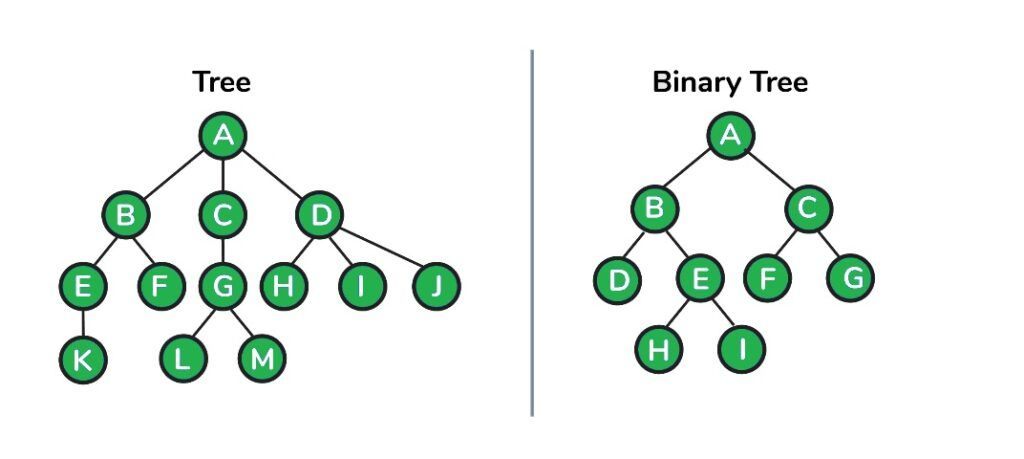#### 25.What is the highest number of nodes that can be found in a binary tree with height k?

• The maximum number of nodes is 2 1-1 where k > 1

#### 26.Write a recursive code to determine the size of a binary tree using Java.

• Think about the fact that every node in an a tree is a member of an entity called Node as shown below:
``` public class Node{
int data;
Node left;
Node right;
}```
• Then the height of the binary tree can be found as follows:
```      int heightOfBinaryTree(Node node)
{
if (node == null)
return 0; // If node is null then height is 0 for that node.
else
{
// compute the height of each subtree
int leftHeight = heightOfBinaryTree(node.left);
int rightHeight = heightOfBinaryTree(node.right);

//use the larger among the left and right height and plus 1 (for the root)
return Math.max(leftHeight, rightHeight) + 1;
}
}```

#### 27. Write Java code to count number of nodes in a binary tree.

```int countNodes(Node root)
{
int count =  1;             //Root itself should be counted
if (root ==null)
return 0;
else
{
count += countNodes(root.left);
count += countNodes(root.right);
return count;
}
}```

#### 28.What is a tree traversal?

• Tree traversal is the process of visiting all nodes in the tree. Since the root (head) is considered to be the initial node, and every node is connected through edges (or links) we always begin with this node. There are three methods we can use to navigate the tree
• Inorder Traversal
• Algorithm:
• Step 1. Explore the left subtree, i.e. Call Inorder(root.left)
• Step 2. Go to the root.
• Step 3. Explore the right subtree, i.e., call Inorder(root.right)
• In order traversal in Java
```   // Print inorder traversal of given tree.
void printInorderTraversal(Node root)
{
if (root == null)
return;

//first traverse to the left subtree
printInorderTraversal(root.left);

//then print the data of node
System.out.print(root.data + " ");

//then traverse to the right subtree
printInorderTraversal(root.right);
}```

Applications in BST, also known as binary search trees (BST) inorder traversal is the process of distributing nodes to ascend order.

Preorder Traversal:

• Algorithm:
• Step 1. Go to the root.
• Step 2. The left subtree is traversed, i.e., call Preorder(root.left)
• Step 3. Find the right subtree to traverse, i.e., call Preorder(root.right)
• Traversal through the preorder in Java:
``` // Print preorder traversal of given tree.
void printPreorderTraversal(Node root)
{
if (root == null)
return;
//first print the data of node
System.out.print(root.data + " ");

//then traverse to the left subtree
printPreorderTraversal(root.left);

//then traverse to the right subtree
printPreorderTraversal(root.right);
}```

Uses:

• Preorder traversal is often employed to create a duplicate of the original tree.
• It can also be used to determine prefix expressions from expression trees.
• Postorder Traversal:
• Algorithm:
• Step 1. Go through the left subtree. i.e. Call Postorder(root.left)
• Step 2. Traverse the right subtree, i.e., call Postorder(root.right)
• Step 3. Find the root.
• Postorder traversal in Java:
```// Print postorder traversal of given tree.
void printPostorderTraversal(Node root)
{
if (root == null)
return;

//first traverse to the left subtree
printPostorderTraversal(root.left);

//then traverse to the right subtree
printPostorderTraversal(root.right);

//then print the data of node
System.out.print(root.data + " ");

}```
• Uses:
• Postorder traversal is often used to remove the tree.
• It’s also beneficial to determine the postfix expression in the expression tree.
• Take the following tree as an example then: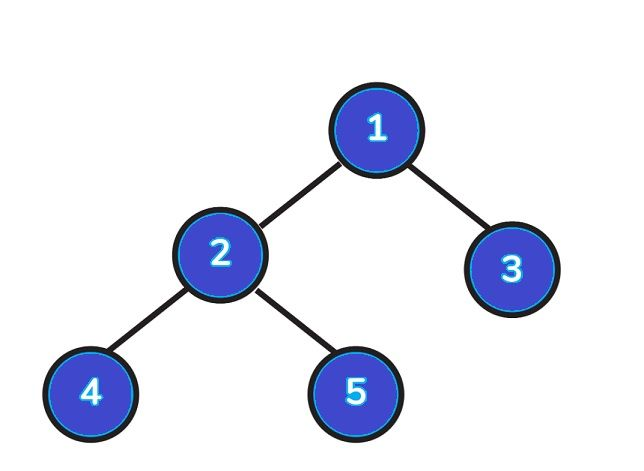• Inorder Traversal => Left, Root, Right : [4, 2, 5, 1, 3]
• Preorder Traversal => Root, Left, Right : [1, 2, 4, 5, 3]
• Postorder Traversal => Left, Right, Root : [4, 5, 2, 3, 1]

#### 29.What is a Binary Search Tree?

• A BST, or binary search tree (BST) is a type of the binary tree structure that stores data in an extremely efficient way, so that nodes in the left-hand sub-tree are lower than that for the node that is at its root as well as the value of nodes to the right of the root are more than the value of the root.
• Furthermore, each of the right and left sub-trees have each their own search tree in any time.

#### 30.What is an AVL Tree?

• The AVL trees act as height-balancing BST. AVL tree inspects the height of both left and right sub-trees, and ensures there is not greater than one. The difference is known as balance Factor and is calculated using. `BalancingFactor is height(left subtree) + height(right subtree)`

#### 31.View of the Print Left side for any Binary tree.

• The primary idea behind solving this issue is to traverse the tree in a an orderly manner and then transfer the information about the level with it. In the event that the level will be being visited for the first time, we save the details for the currently visited node as well as the level currently within the hashmap. We basically get the left-side view by noting the first node in every level.
• When we have completed the traversal it is possible to get the solution simply by walking around the map.
• Take the following tree as an example of how to locate the left-hand view: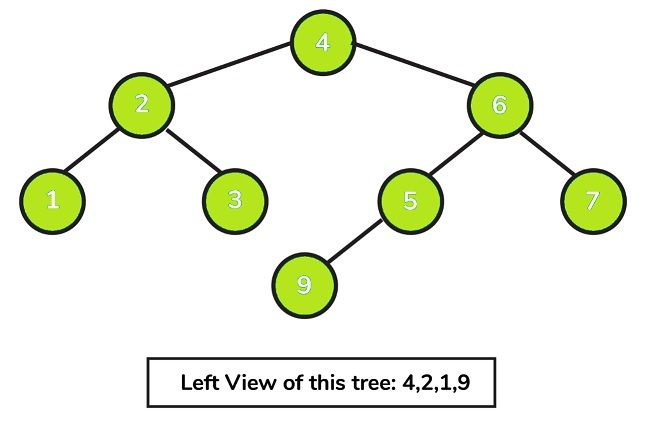#### Left view of a binary tree in Java:

``` import java.util.HashMap;

//to store a Binary Tree node
class Node
{
int data;
Node left = null, right = null;

Node(int data) {
this.data = data;
}
}
public class BinaryTree
{
// traverse nodes in pre-order way
public static void leftViewUtil(Node root, int level, HashMap<Integer, Integer> map)
{
if (root == null) {
return;
}

// if you are visiting the level for the first time
// insert the current node and level info to the map
if (!map.containsKey(level)) {
map.put(level, root.data);
}

leftViewUtil(root.left, level + 1, map);
leftViewUtil(root.right, level + 1, map);
}

// to print left view of binary tree
public static void leftView(Node root)
{
// create an empty HashMap to store first node of each level
HashMap<Integer, Integer> map = new HashMap<>();

// traverse the tree and find out the first nodes of each level
leftViewUtil(root, 1, map);

// iterate through the HashMap and print the left view
for (int i = 0; i <map.size(); i++) {
System.out.print(map.get(i) + " ");
}
}

public static void main(String[] args)
{
Node root = new Node(4);
root.left = new Node(2);
root.right = new Node(6);
root.left.left = new Node(1);
root.left.left = new Node(3);
root.right.left = new Node(5);
root.right.right = new Node(7);
root.right.left.left = new Node(9);

leftView(root);
}
}```

#### 32.What is a graph’s data structure?

A graph is a non-linear data structure which consists of nodes or vertices joined through edges or links the storage of data. The edges connecting the nodes can either be directed, or not.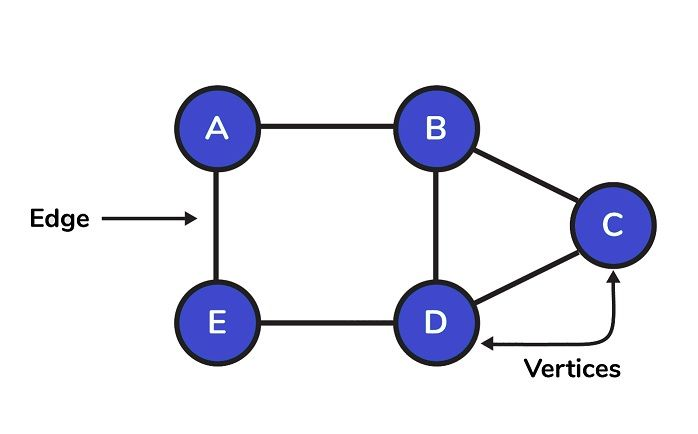#### 33.What are the potential applications to graph structure data structures?

graphs are employed in many variety of applications. Some examples include:

1. Social graphs of networks to understand how information flows within social networks like facebook, linkedin, etc.
2. Neural network graphs where the nodes represent neurons while edges are synapses between them.
3. Transportation grids in which stations are nodes, as well as routes form the edges.
4. Utility graphs for water or power where the vertex points are connections and edges the pipes or wires connecting them.
5. The shortest distance between two end points in the algorithm.

#### 34.How do you present graphs?

We can represent graphs by two methods:

Adjacency matrix is used to represent the representation of data in a sequential fashion.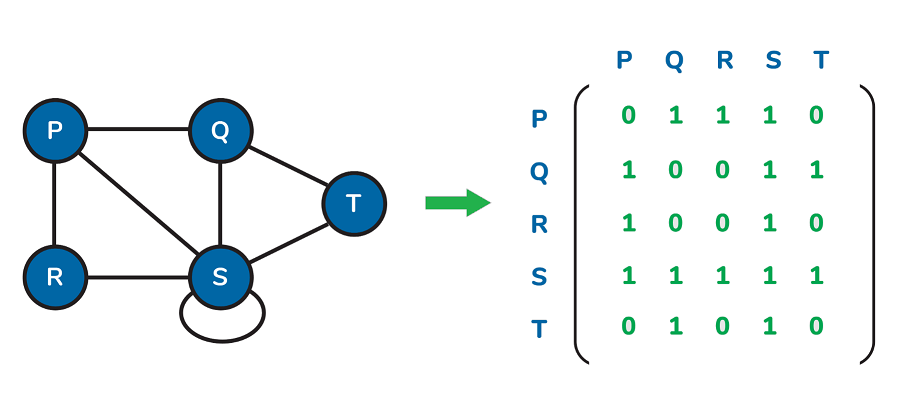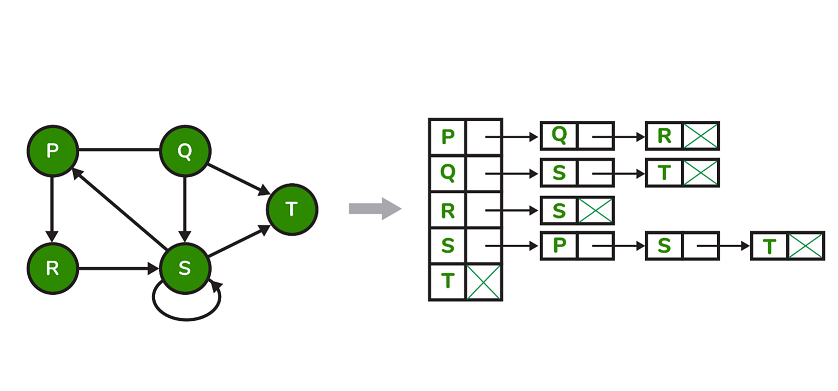#### 35.What is the main difference between tree the graph and tree data structures?

1. Tree and graph differ by the distinction that a tree’s structure is required to be connected and never be looped, while in the graph, there are no limitations.
2. Tree offers insights into the relationship among nodes, in a hierarchical fashion and graph is based on the network model.

#### 36.What is the difference between Breadth First Search (BFS) and Depth First Search (DFS)?

1. BFS or DFS both are methods of traversing a graph. Graph traversal is the procedure of visiting all nodes in the graph.
2. The major distinction the two BFS as well as DFS is the fact that BFS is able to traverse level by level, while DFS follows a route from the beginning to the final node, followed by a second path from the beginning to the end and so on until all nodes have been visited.
3. Additionally, BFS uses queue data structure to store nodes, while DFS makes use of the stack to facilitate the traversal of nodes in implementation.
4. DFS provides more complicated solutions that aren’t ideal, however it does work effectively in cases where the solution is very dense while those solutions offered by BFS are ideal.

#### 37.What is the best way to know the right time to use DFS instead of BFS?

• The use of DFS is heavily dependent on the design of the graph or search tree as well as the location and number of solutions required. Here are some of the most effective scenarios that we could use DFS:
• If you know it is far from the source of the tree then a broad first search (BFS) may be more appropriate.
• In the case that the forest is deep and solutions are scarce deep, a depth-first search (DFS) could take a long time however BFS can be much faster.
• When the trees are extremely large If the tree is very large, a BFS may require excessive memory, and it may not be practical. It is recommended to use DFS in these cases.
• If solutions are common but are located in the forest, we should consider DFS.

#### 38.What exactly is Topological Sorting? the graph?

• The topological sorting process is an linear arrangement of vertices in which for each directed edge that is ij, the vertex i appears ahead of j in the order.
• Topological sorting is possible only for Directed Acyclic graph (DAG).
• Applications:
1. jobs that are scheduled using the dependencies between jobs.
2. Formula cell evaluation ordering in spreadsheets
3. The order of the compilation tasks to be carried out in make files
4. data serialization
5. solving symbol dependencies in linkers.
• Topological Sort Code in Java:
```  // V - total vertices
// visited - boolean array to keep track of visited nodes
// Main Topological Sort Function.
void topologicalSort()
{
Stack<Integer> stack = new Stack<Integer>();

// Mark all the vertices as not visited
boolean visited[] = new boolean[V];
for (int j = 0; j < V; j++){
visited[j] = false;
}
// Call the util function starting from all vertices one by one
for (int i = 0; i < V; i++)
if (visited[i] == false)
topologicalSortUtil(i, visited, stack);

// Print contents of stack -> result of topological sort
while (stack.empty() == false)
System.out.print(stack.pop() + " ");
}

// A helper function used by topologicalSort
void topologicalSortUtil(int v, boolean visited[],
Stack<Integer> stack)
{
// Mark the current node as visited.
visited[v] = true;
Integer i;

// Recur for all the vertices adjacent to the current vertex
Iterator<Integer> it = graph.get(v).iterator();
while (it.hasNext()) {
i = it.next();
if (!visited[i])
topologicalSortUtil(i, visited, stack);
}

// Push current vertex to stack that saves result
stack.push(new Integer(v));
}```

#### 39.If you have an m x n 2D grid of “1’s” that represent land, and ‘0’s which represent water, find an amount of islands (surrounded by water and created by connecting adjacent land in two directions: horizontally or vertically).It is assumed you have the boundaries cases that is, all four edges of the grid are covered by water.

Constraints include:

• M= grid.length
• n = grid[i].length
• 1 = m N= 300
• grid[i][j] is only either ‘0’ or.

Example:
The grid input is:
[“1” , “1” , “1” , “0” , “0”],
[“1” , “1” , “0” , “0” , “0”],
[“0” , “0” , “1” , “0” , “1”],
[“0” , “0” , “0” , “1” , “1”]
]
Output: 3

#### 40.What is a heap-data structure?

Heap is a specific tree-based non-linear structure of data in that the tree forms a full binary tree. The binary tree is believed to be complete when each level is completely filled, with the exception of possibly the final level , and that the final level contains all the elements as much as is left. The two types of heaps are:

1. Max-Heap:
• In a Max-Heap, the data element at the root point must be the most important of all data elements within the tree.
• This property must be applicable to all sub-trees of the binary tree.
2. Min-Heap:
• In a Min-Heap, the data element at the root node has to be the lowest (or minimum) of all the data elements that are present on the trees.
• This property must be applicable to all sub-trees of the binary tree.